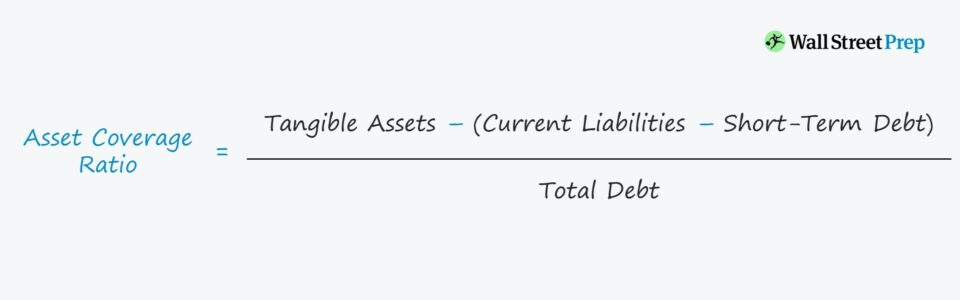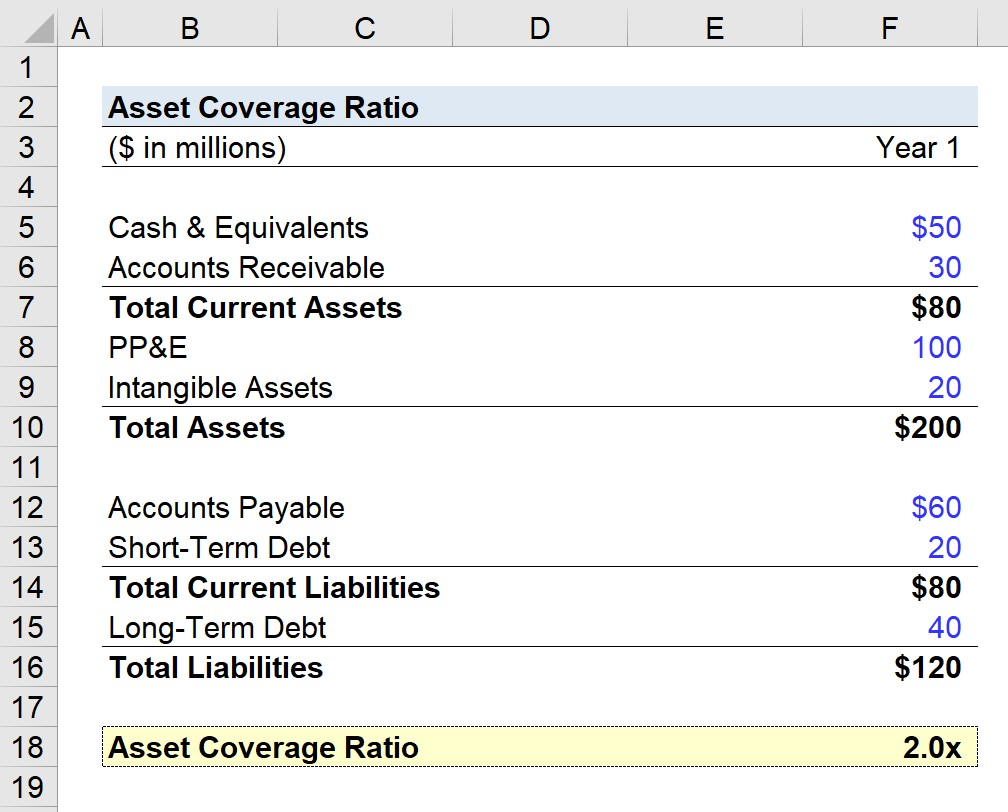# Asset Coverage Ratio

Learn How to Calculate the Asset Coverage Ratio## How to Calculate Asset Coverage Ratio (Step-by-Step)

Higher asset coverage ratios imply lower financial risk associated with the borrower in question.

The asset coverage ratio determines if a company’s liquidated assets can sufficiently cover its debt obligations and liabilities in case its earnings falter unexpectedly.

Ordinarily, earnings and other free cash flow (FCF) metrics are used by lenders to assess the default risk of a potential borrower, as seen in the interest coverage ratio.

However, suppose a company’s earnings are insufficient to meet its required debt obligations (e.g. interest expense, debt amortization).

In that case, a company must resort to selling off its assets to generate enough cash proceeds to avoid defaulting.

Under a worst-case scenario in which the company’s assets would undergo a forced liquidation, the ability for a company’s assets to adequately cover the creditor claims provides more assurance for lenders.

With that said, the asset coverage ratio reflects a “last resort” measure because a forced liquidation scenario implies the borrower has filed for bankruptcy protection.

### The Wharton Online and Wall Street Prep Private Equity Certificate Program

Level up your career with the world's most recognized private equity investing program. Enrollment is open for the May 1 - Jun 25 cohort.

## Asset Coverage Ratio Formula

The formula used to calculate the asset coverage ratio begins by taking the sum of tangible assets and then subtracting current liabilities, excluding short-term debt.

• Asset Coverage Ratio = [(Total Assets – Intangible Assets) – (Current Liabilities – Short-Term Debt)] / Total Debt

Next, the numerator is divided by the total debt balance to arrive at the asset coverage ratio.

The asset coverage ratio represents the number of times that a company can repay its debt using the proceeds from the liquidation of its tangible assets.

However, since intangible assets are subtracted – i.e. non-physical assets that cannot be touched – the remaining asset value is the tangible assets.

Examples of Intangible Assets

• Goodwill
• Intellectual Property (IP)
• Patents
• Customer Lists – i.e. Relationships

The rationale behind leaving out intangible assets from the calculation is that intangibles cannot be easily sold (or even be valued objectively).

Upon deducting intangibles from the calculation of assets, we’re left with only tangible assets, which are physical assets such as:

• Inventory
• Accounts Receivable (A/R)
• Property, Plant & Equipment (PP&E)

The subsequent step is to subtract current liabilities on the numerator, but note that short-term debt is NOT included.

Current liabilities refer to non-financial, short-term obligations such as accounts payable (A/P), which are payments owed to suppliers/vendors.

As for the denominator, the calculation should be straightforward, as it is simply the short-term debt plus long-term debt.

• Short-Term Debt: Matures in <1 Year
• Long-Term Debt: Matures in >1 Year

## Asset Coverage Ratio – Excel Template

We’ll now move to a modeling exercise, which you can access by filling out the form below.Submitting ...

## Asset Coverage Ratio Calculation Example

In our illustrative example, we’ll be using the following model assumptions.

Assets Side:

• Cash & Equivalents = \$50m
• Accounts Receivable = \$30m
• Property, Plant & Equipment = \$100m
• Intangible Assets = \$20m

Liabilities Side:

In Year 1, our company has current assets of \$80m and total assets of \$200m – of which \$20m are from intangible assets.

The tangible assets amount to \$180m (\$200m – \$20m).

On the other side of the balance sheet, our company has \$80m in current liabilities and \$120m in total liabilities, with \$20m in short-term debt and \$40m in long-term debt.

Written out, the formula for calculating the asset coverage ratio is as follows:

• Asset Coverage Ratio = [(\$200m – \$20m) – (\$60m – \$20m)] / (\$40m + \$20m)

Our company’s Year 1 asset coverage comes out to 2.0x.

In other words, if our company’s tangible assets were liquidated and current liabilities were taken care of, the short-term and long-term debt obligations could be paid off twice.

To reiterate from earlier, the higher the asset coverage ratio, the less risk there is for the company (i.e. the borrower has sufficient proceeds post-liquidation to cover its outstanding debt), so our company appears to be financially sound.Step-by-Step Online Course

### Everything You Need To Master Financial Modeling

Enroll in The Premium Package: Learn Financial Statement Modeling, DCF, M&A, LBO and Comps. The same training program used at top investment banks.Inline FeedbacksMSIKUMAR
March 18, 2022 11:38 am

Very good informative.';
Learn Financial Modeling Online

Everything you need to master financial and valuation modeling: 3-Statement Modeling, DCF, Comps, M&A and LBO.

X

The Wall Street Prep Quicklesson Series

7 Free Financial Modeling Lessons

Get instant access to video lessons taught by experienced investment bankers. Learn financial statement modeling, DCF, M&A, LBO, Comps and Excel shortcuts.